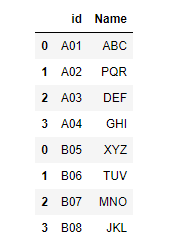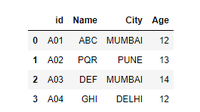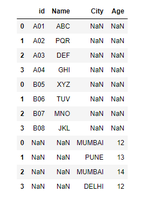# How to combine two dataframe in Python – Pandas?

• Difficulty Level : Expert
• Last Updated : 02 Dec, 2020

Prerequisites: Pandas

In many real-life situations, the data that we want to use comes in multiple files. We often have a need to combine these files into a single DataFrame to analyze the data. Pandas provide such facilities for easily combining Series or DataFrame with various kinds of set logic for the indexes and relational algebra functionality in the case of join / merge-type operations. In addition, pandas also provide utilities to compare two Series or DataFrame and summarize their differences.

## Concatenating DataFrames

The concat() function in pandas is used to append either columns or rows from one DataFrame to another. The concat() function does all the heavy lifting of performing concatenation operations along an axis while performing optional set logic (union or intersection) of the indexes (if any) on the other axes.

## Python3

 `import` `pandas as pd ` `# First DataFrame ` `df1 ``=` `pd.DataFrame({``'id'``: [``'A01'``, ``'A02'``, ``'A03'``, ``'A04'``], ` `                    ``'Name'``: [``'ABC'``, ``'PQR'``, ``'DEF'``, ``'GHI'``]}) ` ` `  `# Second DataFrame ` `df2 ``=` `pd.DataFrame({``'id'``: [``'B05'``, ``'B06'``, ``'B07'``, ``'B08'``], ` `                    ``'Name'``: [``'XYZ'``, ``'TUV'``, ``'MNO'``, ``'JKL'``]}) ` ` `  ` `  `frames ``=` `[df1, df2] ` ` `  `result ``=` `pd.concat(frames) ` `display(result) `

Output:## Joining DataFrames

When we concatenated our DataFrames we simply added them to each other i.e. stacked them either vertically or side by side. Another way to combine DataFrames is to use columns in each dataset that contain common values (a common unique id). Combining DataFrames using a common field is called “joining”. The columns containing the common values are called “join key(s)”. Joining DataFrames in this way is often useful when one DataFrame is a “lookup table” containing additional data that we want to include in the other.

Note: This process of joining tables is similar to what we do with tables in an SQL database.

When gluing together multiple DataFrames, you have a choice of how to handle the other axes (other than the one being concatenated). This can be done in the following two ways :

• Take the union of them all, join=’outer’. This is the default option as it results in zero information loss.
• Take the intersection, join=’inner’.

Example:

## Python3

 `import` `pandas as pd ` ` `  `df1 ``=` `pd.DataFrame({``'id'``: [``'A01'``, ``'A02'``, ``'A03'``, ``'A04'``], ` `                    ``'Name'``: [``'ABC'``, ``'PQR'``, ``'DEF'``, ``'GHI'``]}) ` ` `  `df3 ``=` `pd.DataFrame({``'City'``: [``'MUMBAI'``, ``'PUNE'``, ``'MUMBAI'``, ``'DELHI'``], ` `                    ``'Age'``: [``'12'``, ``'13'``, ``'14'``, ``'12'``]}) ` ` `  `# the default behaviour is join='outer' ` `# inner join ` ` `  `result ``=` `pd.concat([df1, df3], axis``=``1``, join``=``'inner'``) ` `display(result) `

Output:## Concatenating using append

A useful shortcut to concat() is append() instance method on Series and DataFrame. These methods actually predated concat.

Example:

## Python3

 `import` `pandas as pd ` `# First DataFrame ` `df1 ``=` `pd.DataFrame({``'id'``: [``'A01'``, ``'A02'``, ``'A03'``, ``'A04'``], ` `                    ``'Name'``: [``'ABC'``, ``'PQR'``, ``'DEF'``, ``'GHI'``]}) ` ` `  `# Second DataFrame ` `df2 ``=` `pd.DataFrame({``'id'``: [``'B05'``, ``'B06'``, ``'B07'``, ``'B08'``], ` `                    ``'Name'``: [``'XYZ'``, ``'TUV'``, ``'MNO'``, ``'JKL'``]}) ` ` `  `# append method ` `result ``=` `df1.append(df2) ` `display(result) `

Output:Note: append() may take multiple objects to concatenate.

Example:

## Python3

 `import` `pandas as pd ` `# First DataFrame ` `df1 ``=` `pd.DataFrame({``'id'``: [``'A01'``, ``'A02'``, ``'A03'``, ``'A04'``], ` `                    ``'Name'``: [``'ABC'``, ``'PQR'``, ``'DEF'``, ``'GHI'``]}) ` ` `  `# Second DataFrame ` `df2 ``=` `pd.DataFrame({``'id'``: [``'B05'``, ``'B06'``, ``'B07'``, ``'B08'``], ` `                    ``'Name'``: [``'XYZ'``, ``'TUV'``, ``'MNO'``, ``'JKL'``]}) ` ` `  `df3 ``=` `pd.DataFrame({``'City'``: [``'MUMBAI'``, ``'PUNE'``, ``'MUMBAI'``, ``'DELHI'``], ` `                    ``'Age'``: [``'12'``, ``'13'``, ``'14'``, ``'12'``]}) ` ` `  ` `  `# appending multiple DataFrame ` `result ``=` `df1.append([df2, df3]) ` `display(result) `

Output:My Personal Notes arrow_drop_up
Recommended Articles
Page :